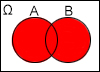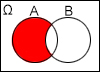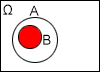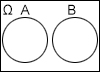# Events, Operations with events, Complete system of events

## Events

A is a subset of Ω-sample space

Impossible event

$\varnothing$

Sure event

$\Omega$

## Operations with events

The event that occures, when either A or B or both occur.
$A\cup B=A+B$The event that occures, when both A and B occur simultaneously.

$A\cap B=A·B$The event that occures, when A accumres, but B not.
$A\setminus B$The event that occures, when A does not occure.

$\overline{A}$B event entails A event.

$B\subset A$Events A end B are mutually exclusive events.## Complete system of events

$\sum _{i=1}^{n}{A}_{i}={A}_{1}+{A}_{2}+{A}_{3}+...+{A}_{n}=\Omega$

Two events can not happen simultaneously.

Keywords: Events, Operations with events, Complete system of events